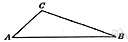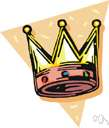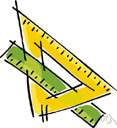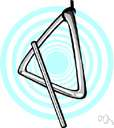# triangle

(redirected from carotid triangle, inferior)
Also found in: Thesaurus, Medical, Financial, Encyclopedia.
Related to carotid triangle, inferior: carotid body, external carotid artery, carotid sheath

## tri·an·gle

(trī′ăng′gəl)
n.
1.
a. The plane figure formed by connecting three points not in a straight line by straight line segments; a three-sided polygon.
b. Something shaped like such a figure: a triangle of land.
2. Any of various flat, three-sided drawing and drafting guides, used especially to draw straight lines at specific angles.
3. Music A percussion instrument consisting of a piece of metal in the shape of a triangle open at one angle.
4. A relationship involving three people, especially a ménage à trois.

[Middle English, from Old French, from Latin triangulum, from neuter of triangulus, three-angled : tri-, tri- + angulus, angle.]

## triangle

(ˈtraɪˌæŋɡəl)
n
1. (Mathematics) geometry a three-sided polygon that can be classified by angle, as in an acute triangle, or by side, as in an equilateral triangle. Sum of interior angles: 180°; area: base × height
2. any object shaped like a triangle
3. any situation involving three parties or points of view. See also eternal triangle
4. (Instruments) music a percussion instrument consisting of a sonorous metal bar bent into a triangular shape, beaten with a metal stick
5. a group of three
[C14: from Latin triangulum (noun), from triangulus (adjective), from tri- + angulus corner]
ˈtriˌangled adj

## tri•an•gle

art at tribrach
(ˈtraɪˌæŋ gəl)

n.
1. a closed plane figure having three sides and three angles.
2. a flat triangular piece with straight edges, used in connection with a T square for drawing perpendicular lines, geometric figures, etc.
3. any three-cornered or three-sided figure, object, or piece: a triangle of land.
4. a musical percussion instrument that consists of a steel triangle, open at one corner, that is struck with a steel rod.
5. a group of three; triad.
6. a situation involving three persons, esp. one in which two of them are in love with the third.
[1350–1400; Middle English < Latin triangulum, n. use of neuter of triangulus three-cornered. See tri-, angle1]
tri′an`gled, adj.

## tri·an·gle

(trī′ăng′gəl)
A closed geometric figure consisting of three sides.

## Triangle

a set of three.
Example: long wavering triangle of waterfowl, 1895.
ThesaurusAntonymsRelated WordsSynonymsLegend:
 Noun 1triangle - a three-sided polygon    polygon, polygonal shape - a closed plane figure bounded by straight sidesacute triangle, acute-angled triangle - a triangle whose interior angles are all acuteequiangular triangle, equilateral triangle - a three-sided regular polygonisosceles triangle - a triangle with two equal sidesoblique triangle - a triangle that contains no right angleobtuse triangle, obtuse-angled triangle - a triangle that contains an obtuse interior angleright triangle, right-angled triangle - a triangle with one right anglescalene triangle - a triangle with no two sides of equal lengthcuneus, wedge, wedge shape - any shape that is triangular in cross section 2triangle - something approximating the shape of a triangle; "the coastline of Chile and Argentina and Brazil forms two legs of a triangle"shape, form - the spatial arrangement of something as distinct from its substance; "geometry is the mathematical science of shape" 3Triangle - a small northern constellation near Perseus between Andromeda and AriesTriangulum 4triangle - any of various triangular drafting instruments used to draw straight lines at specified anglesdrafting instrument - an instrument used by a draftsman in making drawings 5triangle - a percussion instrument consisting of a metal bar bent in the shape of an open trianglepercussion instrument, percussive instrument - a musical instrument in which the sound is produced by one object striking another
Translations
مُثَلَّثمُثَلَّث موسيقي
triangltrojúhelník
trekanttriangel
triangulo
kolmiotriangeli
trokuttriangl
háromszögtriangulum
òríhornòríhyrningurþríhornþríhyrningur
トライアングル三角形
삼각형트라이앵글
triangulum
trikampis
trijstūris
triangel
trikotniktriangel
triangeltrehörningtrekant
กิ๋ง เครื่องดนตรีรูปสามเหลี่ยมสามเหลี่ยม
üçgentriangelüç köşe
hình tam giáckẻng ba gốc

## triangle

[ˈtraɪæŋgl] N (also Mus) →

[ˈtraɪæŋgəl] n
(MATHEMATICS)
(MUSIC)

## triangle

nDreieck nt; (= set square)(Zeichen)dreieck nt; (Mus) → Triangel m; (fig: = relationship) → Dreiecksbeziehung f

## triangle

[ˈtraɪˌæŋgl] n (Math, Mus) → triangolo

## triangle

(ˈtraiӕŋgl) noun
1. a two-dimensional figure with three sides and three angles.
2. a musical instrument consisting of a triangular metal bar that is struck with a small hammer.
triˈangular (-gju-) adjective
in the shape of a triangle. a triangular road-sign; It is triangular in shape.

## triangle

triangl, trojúhelník trekant, triangel kolmio, triangeli triangl, trokut トライアングル, 三角形 삼각형, 트라이앵글 triangel กิ๋ง เครื่องดนตรีรูปสามเหลี่ยม, สามเหลี่ยม hình tam giác, kẻng ba gốc

## tri·an·gle

n. triángulo.
Site: Follow: Share:
Open / Close